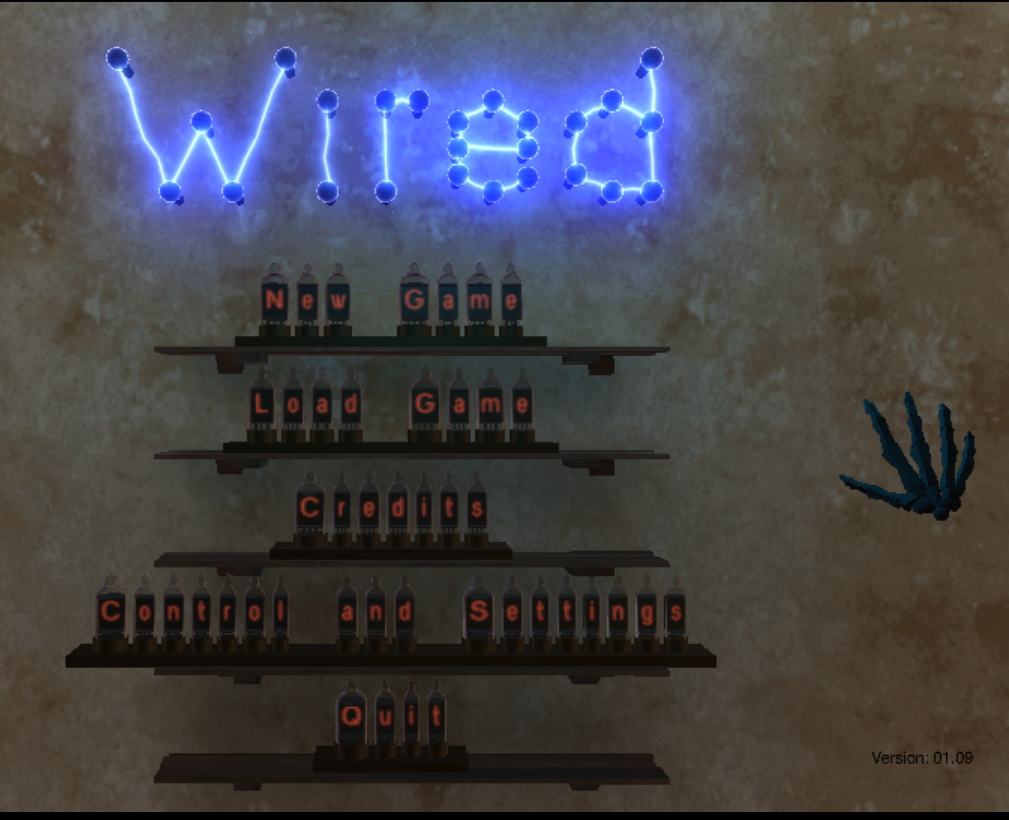MHS Library | Science

# Introduction to circuits and Ohm's law

Ohm’s Law is V = IR, where V = voltage, I = current, and R = resistance. Ohm’s Law allows you to determine characteristics of a circuit, such as how much current is flowing through it, if you know the voltage of the battery in the circuit and how much resistance is in the circuit. Created by Sal Khan.

## Games

WIRED - A platform based game that requires you to build circuits. Starts very basic and gets more complex as you travel through the game.gamify